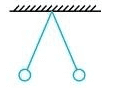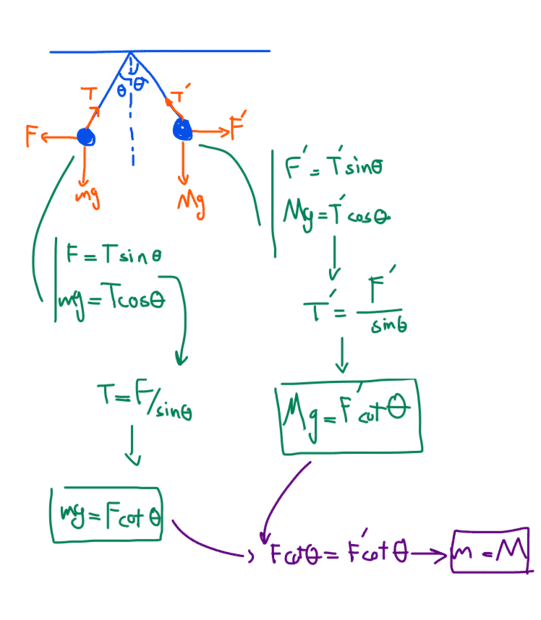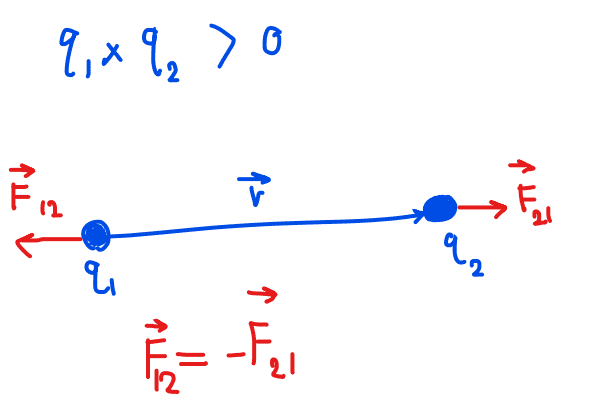# Balance of forces for two charged spheres hanging from the ceiling

• MatinSAR

#### MatinSAR

Homework Statement
I know that in order for the ropes to have an equal angle as shown in the figure below, the weight of the two balls and the sign of the electric charge must be the same.
But how about the amount of charge?
Relevant Equations
F=kqQ/(r^2)I think if we don't consider electron's/proton's mass then we can say that the amount of charge doesn't need to be equal according to Newton's 3rd Law. I mean having q on one ball and 2q on another ball , still makes the angles having the same size. Is it true ?

What if we consider proton's mass(or electron's mass) ???
I think both balls should have same amount of electric charge and number of protons(electrons)on each ball should be equal.

If I understand you, you are concerned about the change in mass resulting from the charge that a ball may be holding.
The givens are that the ropes are at equal angles and there is no other force applied to the balls (for example, between a ball and the ceiling).

If there is no other context to this question, I would explicitly invoke massless electrons - from the same source as the massless ropes commonly used in problems such as these - or from the same source as a ceiling that applies no electrostatic force to the hanging balls.

•MatinSAR
I know that in order for the ropes to have an equal angle as shown in the figure below, the weight of the two balls and the sign of the electric charge must be the same.
Are you sure about the bold part? I certainly agree that the charges must have the same sign but are you sure there is no way to fiddle the mass/charge ratio so that the weights are not the same?

•MatinSAR
Relevant Equations:: F=kqQ/(r^2)

I think if we don't consider electron's/proton's mass ...
I do not see the electron mass or the proton mass in your relevant formula.
But I don't see an expression for each of the two angles either, so I wonder how you can make any statements at all ?

•MatinSAR
Homework Statement:: I know that in order for the ropes to have an equal angle as shown in the figure below, the weight of the two balls and the sign of the electric charge must be the same.
But how about the amount of charge?
Consider two facts that you must already be familiar with.
1. For the two masses to hang in equilibrium, their center of mass must be below the level of support.
2. The force that charge A exerts on charge B has the same magnitude and opposite direction to the force that B exerts on A.
Put the two together.

•MatinSAR
If I understand you, you are concerned about the change in mass resulting from the charge that a ball may be holding.
The givens are that the ropes are at equal angles and there is no other force applied to the balls (for example, between a ball and the ceiling).

If there is no other context to this question, I would explicitly invoke massless electrons - from the same source as the massless ropes commonly used in problems such as these - or from the same source as a ceiling that applies no electrostatic force to the hanging balls.
Good idea. Thank you for your time.
Are you sure about the bold part? I certainly agree that the charges must have the same sign but are you sure there is no way to fiddle the mass/charge ratio so that the weights are not the same?
I think they should have similar mass.I do not see the electron mass or the proton mass in your relevant formula.
But I don't see an expression for each of the two angles either, so I wonder how you can make any statements at all ?
I said the charges should have same sign because otherwise they will get closer.
And I said they should have equal mass because of above image.
I was not sure about the amount of charge on each ball ...
Consider two facts that you must already be familiar with.
1. For the two masses to hang in equilibrium, their center of mass must be below the level of support.
2. The force that charge A exerts on charge B has the same magnitude and opposite direction to the force that B exerts on A.
Put the two together.
Can't we find out if amount of electric charge on each ball should be the same or not without fact number 1?

•BvU
I think they should have similar mass.
So, you've moved from identical mass to "similar" mass. Even for that, I have to ask ... why?

•MatinSAR
So, you've moved from identical mass to "similar" mass. Even for that, I have to ask ... why?
What is the difference ???

What is the difference ???
?? You don't understand the difference between "identical" and "similar"

•MatinSAR
?? You don't understand the difference between "identical" and "similar"
I mean m1=m2 ...

Hello @MatinSAR. I follow your work shown in green in your figure of post #6. Can you state your reasoning for writing the purple equation ##F \cot \theta = F' \cot \theta##? I think you're probably thinking correctly and you're getting this equation from something you mentioned in your first post. But please confirm how you got the purple equation.

You were also asking whether the two charges had to have the same magnitude. You should be able to answer this by inspecting Coulomb's law.

As @.Scott mentioned, changing the charge of a ball does not change the mass of the ball by any significant amount.

Last edited:
•MatinSAR
I mean m1=m2 ...
Which is not necessary for this problem --- that's what I'm trying to get you to see.

•MatinSAR
I think you're probably thinking correctly and you're getting this equation from something you mentioned in your first post. But please confirm how you got the purple equation.
Hello. Because of Newton's 3rd law F should be equal to F'.You were also asking whether the two charges had to have the same magnitude. You should be able to answer this by inspecting Coulomb's law.
I think it's not necessary because of the fact that F12=F21.
As @.Scott mentioned, changing the charge of a ball does not change the mass of the ball by any significant amount.
So mass of the balls should be the same and the charges should have same sign ...
And it is not necessary to have same amount of charge on each ball.
Is it true?

Which is not necessary for this problem --- that's what I'm trying to get you to see.
Can you tell me why ?!
Here #6 I have proved that the mass of two balls should be equal.(m1= m2)

Can you tell me why ?!
Here #6 I have proved that the mass of two balls should be equal.(m1= m2)
No, you did not. You proved that if you assume the charges are identical then the masses have to be identical. That is a vacuous truth. Please THINK about the problem. You clearly did not think through post #3.

•MatinSAR
Hello. Because of Newton's 3rd law F should be equal to F'.
Yes. The 3rd law is the basis for your purple equation.

I think it's not necessary because of the fact that F12=F21.
OK. But, also, the electric force depends on the charges as the product of the charges. So, the electric force will not change if you change the individual charges such that their product remains the same.

So mass of the balls should be the same and the charges should have same sign ...
And it is not necessary to have same amount of charge on each ball.
Is it true?
Yes.

•MatinSAR
No, you did not. You proved that if you assume the charges are identical then the masses have to be identical. That is a vacuous truth. Please THINK about the problem. You clearly did not think through post #3.
Thank you for your help and time. I'll spend more time on it tomorrow morning ... perhaps I will understand what were you trying to tell me ...Yes. The 3rd law is the basis for your purple equation.

OK. But, also, the electric force depends on the charges as the product of the charges. So, the electric force will not change if you change the individual charges such that their product remains the same.

Yes.
I have understand it completely.
Thanks a lot for your time.

You proved that if you assume the charges are identical then the masses have to be identical.
No, the assumption made in post #6 is that the electrostatic forces are equal and opposite. Seems fair.

•MatinSAR
No, the assumption made in post #6 is that the electrostatic forces are equal and opposite. Seems fair.
Good point. I was thinking about it incorrectly.

•MatinSAR